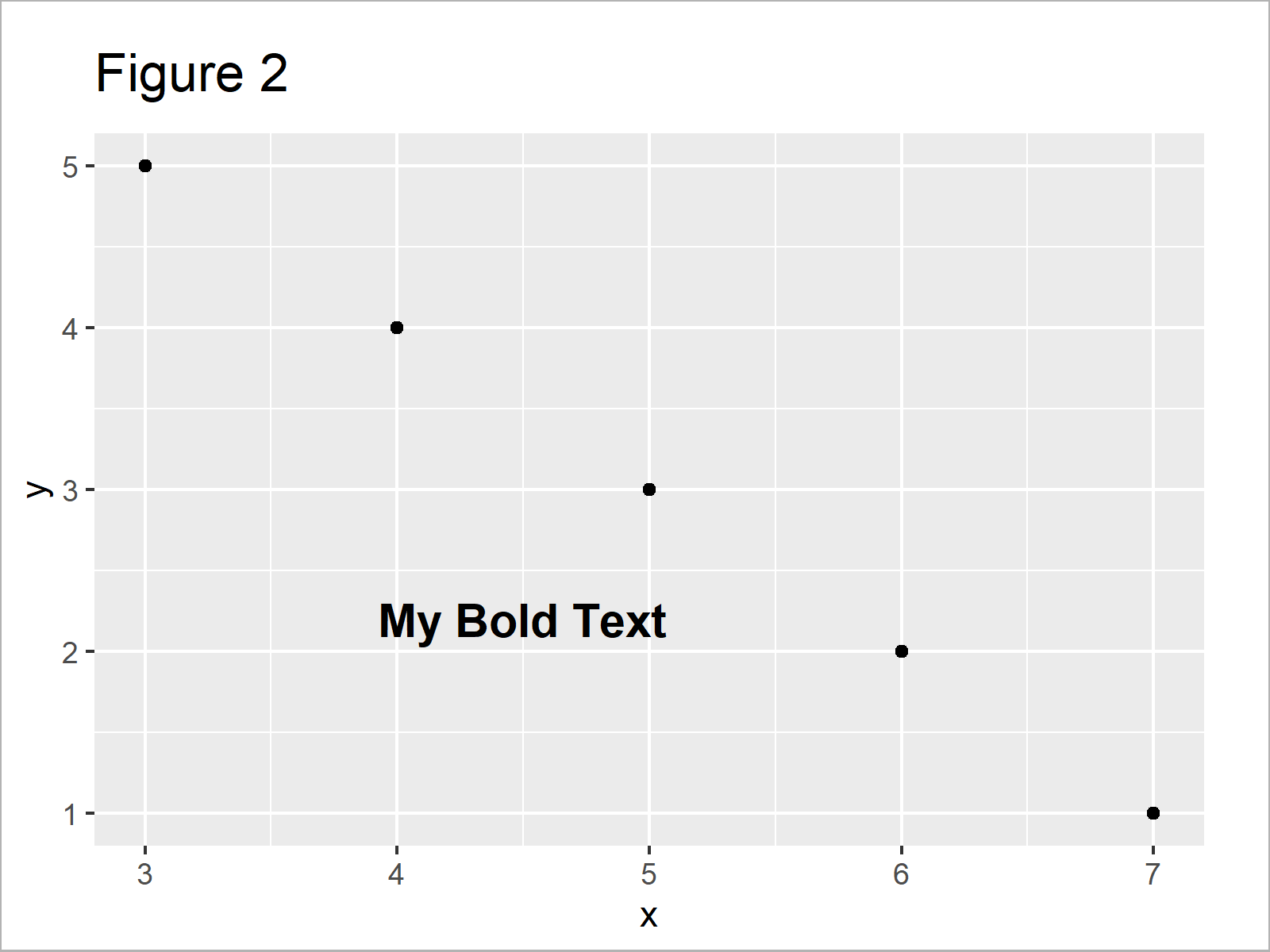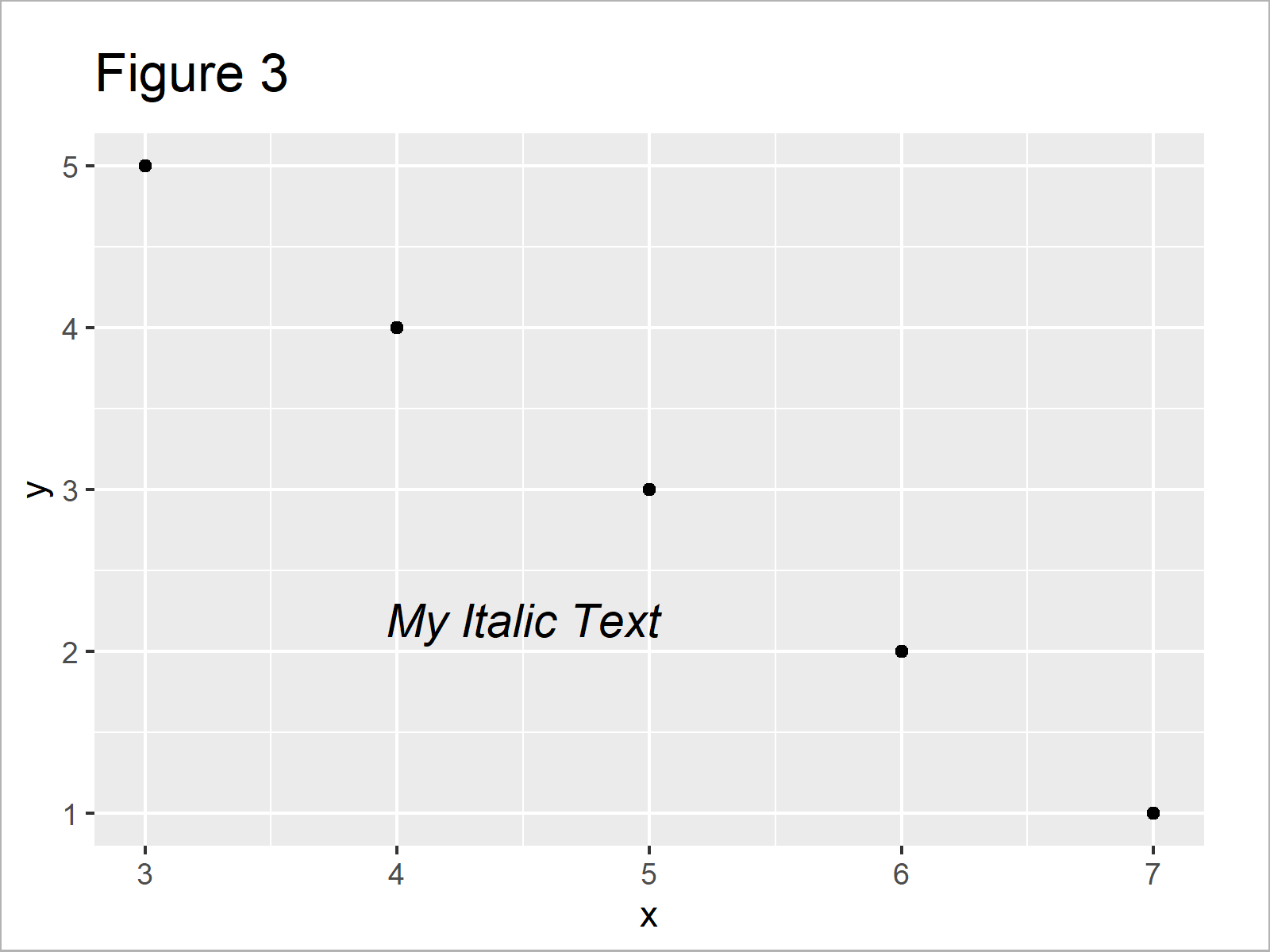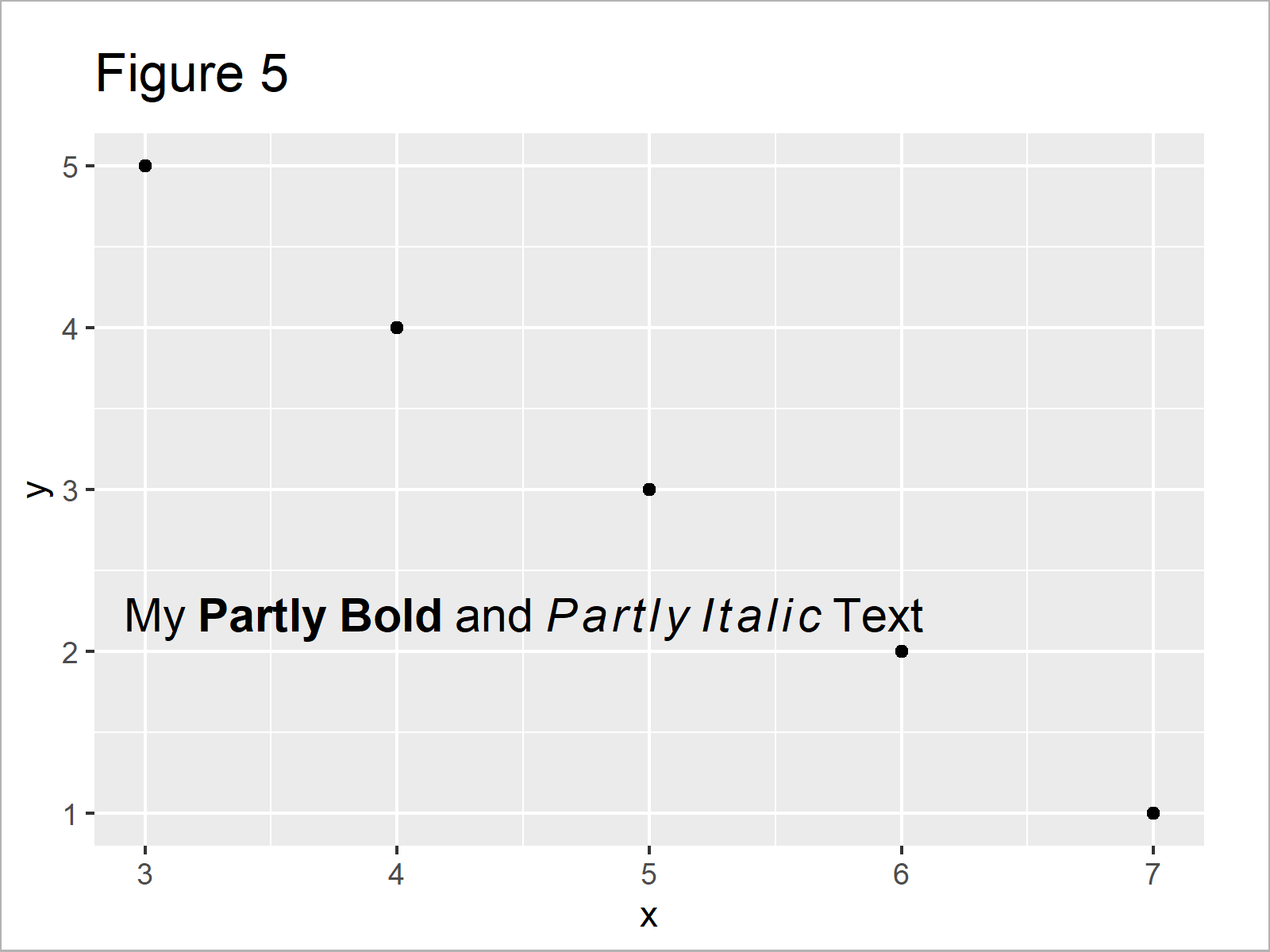# Add Bold & Italic Text to ggplot2 Plot in R (4 Examples)

In this tutorial you’ll learn how to bold and italic text elements to a ggplot2 graph in the R programming language.

The content of the article looks as follows:

It’s time to dive into the examples!

## Example Data, Packages & Basic Plot

Consider the following example data:

```data <- data.frame(x = 7:3,         # Create example data
y = 1:5)
data                                # Print example data```Table 1 shows the structure of our example data – It has five rows and two columns.

We also need to install and load the ggplot2 package, if we want to use the corresponding functions:

```install.packages("ggplot2")         # Install ggplot2 package

Now, we can plot our data as follows:

```ggp <- ggplot(data, aes(x, y)) +    # Create ggplot2 plot
geom_point()
ggp                                 # Draw ggplot2 plot```After executing the previous R code the ggplot2 scatterplot without text elements visualized in Figure 1 has been created.

## Example 1: Annotate Bold Text Element to ggplot2 Plot

This example illustrates how to draw a bold text element to a ggplot2 graph in R.

For this, we have to specify the fontface argument within the annotate function to be equal to “bold”:

```ggp +                               # Add bold text element to plot
annotate("text", x = 4.5, y = 2.2, size = 5,
label = "My Bold Text",
fontface = "bold")```As you can see in Figure 2, we have created a ggplot2 plot containing a bold text element with the previous code.

## Example 2: Annotate Italic Text Element to ggplot2 Plot

Example 2 illustrates how to add cursive text elements to a ggplot2 graph by specifying the fontface argument to be equal to “italic”:

```ggp +                               # Add italic text element to plot
annotate("text", x = 4.5, y = 2.2, size = 5,
label = "My Italic Text",
fontface = "italic")```Figure 3 shows the output of the previous R syntax: A ggplot2 plot with italic text.

## Example 3: Annotate Bold & Italic Text Element to ggplot2 Plot

The following R code shows how to make the text in a ggplot2 plot bold AND italic by specifying multiple styles to fontface (i.e. fontface = “bold.italic”):

```ggp +                               # Add bold and italic text element to plot
annotate("text", x = 4.5, y = 2.2, size = 5,
label = "My Bold and Italic Text",
fontface = "bold.italic")```As shown in Figure 4, the previously shown R programming syntax has created a plot with a text element in bold and italic.

## Example 4: Annotate Partly Bold & Partly Italic Text Element to ggplot2 Plot

So far, we have changed the style of entire text elements. However, it is also possible to set only specific parts of a text element to bold or italic.

This example explains how to use the plotmath syntax and the parse argument to make some parts of our text bold and other parts italic.

Consider the following R code:

```ggp +                               # Add partly bold/italic text element to plot
annotate("text", x = 4.5, y = 2.2, size = 5,
label = "My~bold(Partly~Bold)~and~italic(Partly~Italic)~Text",
parse = TRUE)```After executing the previous syntax the xy-plot shown in Figure 5 has been plotted. Some symbols of the text are bold and other parts are italic

## Video & Further Resources

Would you like to learn more about ggplot2 plots? Then I recommend having a look at the following video of my YouTube channel. In the video, I illustrate the R programming codes of this article:

Please accept YouTube cookies to play this video. By accepting you will be accessing content from YouTube, a service provided by an external third party.If you accept this notice, your choice will be saved and the page will refresh.

In addition, you may want to have a look at some of the other tutorials on my website. You can find a selection of tutorials about related topics such as graphics in R, regression models, ggplot2, and text elements below:

You have learned in this tutorial how to annotate bold and italic text to a ggplot2 graphic in R. In case you have additional questions, let me know in the comments.

Subscribe to the Statistics Globe Newsletter